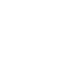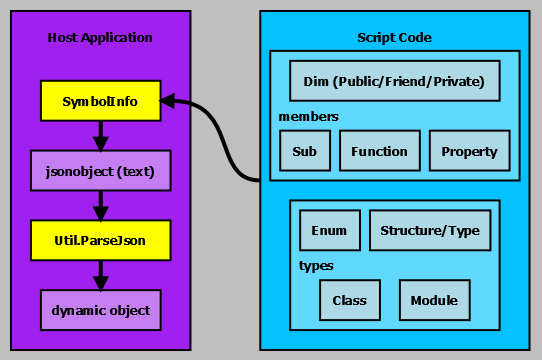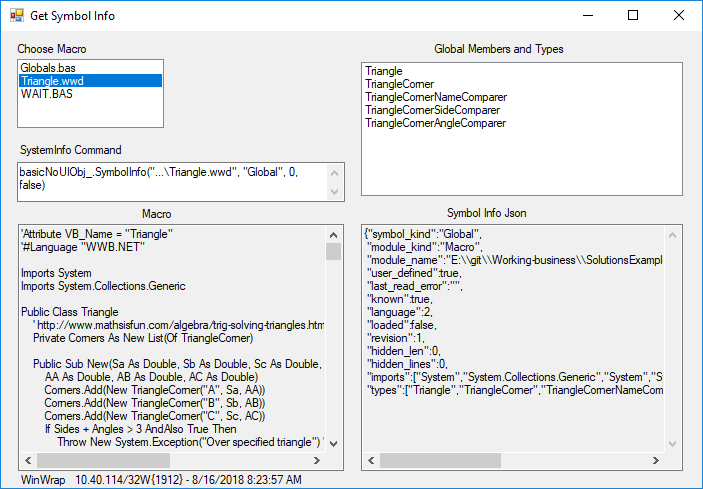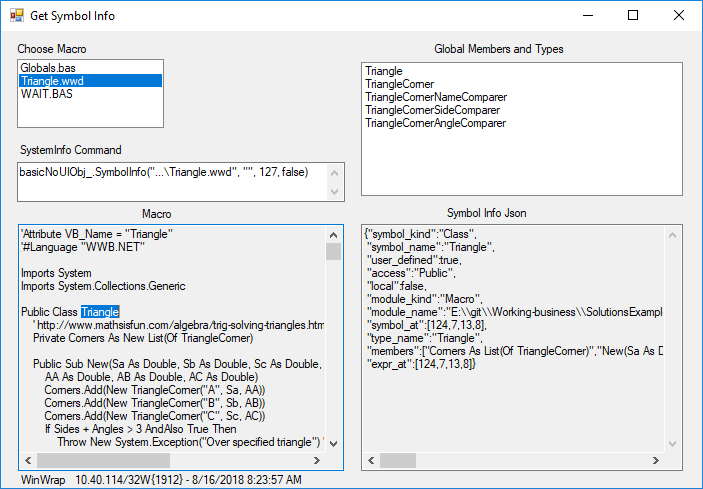An error occurred in script '/home/yhr1ef23xez5/public_html/web2/app/model/bannerhit.php' on line 17:
Undefined index: HTTP_REFERER

```Array
(
[type] => solution
[name] => symbol-info
)
```

WinWrap® | Symbol InformationWinWrap®

# Symbol Information

WinWrap® Basic is an embedded macro language component available for .NET and COM 32/64 bit Windows applications. The WinWrap® Basic Component is an alternative to Visual Basic for Applications (VBA), ActiveX (e.g. VBScript, JScript, PerlScript, Rexx-based WSH engines and others), and VSTA for this purpose. The WinWrap® Basic Component is compatible with VBA, Sax Basic, VB.NET and Visual Basic 6.0 style scripts.

### Symbol Information

• Script, Module or Project does not need to be parsed for execution
• Get Global Methods, Structures, Types, Modules, Classes and Enums
• Get Members of Scripts, Structures, Types, Modules, Classes and Enums
• Return identifier definition location
• Determine expression result type
• Supports all scripting languages (WWB-COM, WWB.NET and WWB.NET/Compiled)### Symbol Information

WinWrap® Basic treats script code as structured collection of symbols. The SymbolInfo method allows an application to determine what's in a script before execution. For this solution we will explore the symbols defined by the Triangle.wwd script. The source code for this solution is available for download here.

The triangle.wwd macro code.

'Attribute VB_Name = "Triangle" '#Language "WWB.NET" Imports System Imports System.Collections.Generic Public Class Triangle ' http://www.mathsisfun.com/algebra/trig-solving-triangles.html Private Corners As New List(Of TriangleCorner) Public Sub New(Sa As Double, Sb As Double, Sc As Double, AA As Double, AB As Double, AC As Double) Corners.Add(New TriangleCorner("A", Sa, AA)) Corners.Add(New TriangleCorner("B", Sb, AB)) Corners.Add(New TriangleCorner("C", Sc, AC)) If Sides + Angles > 3 AndAlso True Then Throw New System.Exception("Over specified triangle") ' End If End Sub Public Function Angle(index As Integer) As Double Return Corners(index).Angle End Function Public Function Side(index As Integer) As Double Return Corners(index).Side End Function Public Function Solve() Dim n As Integer Do n = Sides + Angles If Sides = 3 Then TrySSS() If Sides = 2 AndAlso Angles >= 1 Then TrySAS() If Sides = 2 AndAlso Angles >= 1 Then TrySSA() If Sides = 1 AndAlso Angles >= 2 Then TryAAS() If Angles = 2 Then TryAA() Loop While Not Solved AndAlso Sides + Angles > n ' continue while making progress SortNames() Return Me End Function Public ReadOnly Property Solved As Boolean Get Return Sides = 3 AndAlso Angles = 3 End Get End Property Private Sub TrySSA() SortAngles() If Side(2) <> 0 AndAlso Angle(1) = 0 Then ' Law of Sines: a/sin(A) = b/sin(B) = c/sin(C) ' solve for B: sin(B)/b = sin(C)/c ' solve for B: B = asin(sin(C)*b/c) ' side b is Side(1) ' side c is Side(2) ' angle C is Angle(2) Dim asin = Math.Sin(Angle(2)) * Side(1) / Side(2) ' cope with asin slightly out of range If asin < -1 Then asin = -1 If asin > 1 Then asin = 1 Corners(1).Angle = Math.Asin(asin) End If End Sub Private Sub TrySAS() SortSides() If Angle(0) <> 0 AndAlso Side(0) = 0 Then ' Law of Cosines: a^2 = b^2 + c^2 - 2*b*c*cos(A) ' solve for a: a = sqrt(b^2 + c^2 - 2*b*c*cos(A)) ' angle A is Angle(0) ' side b is Side(1) ' side c Side(2) ' side a is Side(0) Corners(0).Side = Math.Sqrt(Side(1) ^ 2 + Side(2) ^ 2 - 2 * Side(1) * Side(2) * Math.Cos(Angle(0))) End If End Sub Private Sub TryAA() SortAngles() If Angle(0) = 0 Then ' Law of Angles: A + B + C = 180 ' solve for A: A = 180 - B - C ' angle A is Angle(0) ' angle B is Angle(1) ' angle C is Angle(2) Corners(0).Angle = Math.PI - Angle(1) - Angle(2) End If End Sub Private Sub TryAAS() SortSides() If Side(1) = 0 Then SortAngles() ' Law of Sines: a/sin(A) = b/sin(B) = c/sin(C) ' solve for b: b = c*sin(B)/sin(C) ' side b is Side(1) ' angle B is Angle(1) ' side c is Side(2) ' angle C is Angle(2) Corners(1).Side = Side(2) * Math.Sin(Angle(1)) / Math.Sin(Angle(2)) End If End Sub Private Sub TrySSS() SortAngles() If Angle(0) = 0 Then ' Law of Cosines: a^2 = b^2 + c^2 - 2*b*c*cos(A) ' solve for A: A = acos((b^2 + c^2 - a^2)/(2*b*c)) ' side a is Side(0) ' side b is Side(1) ' side c is Side(2) ' angle A is Angle(0) Corners(0).Angle = Math.Acos((Side(1) ^ 2 + Side(2) ^ 2 - Side(0) ^ 2) / (2 * Side(1) * Side(2))) 'If Double.IsNaN(Corners(0).Angle) Then 'Throw New System.Exception("Sides can not make a triangle.") 'End If End If End Sub Private Sub SortNames() Dim tc As New TriangleCornerNameComparer Corners.Sort(tc) End Sub Private Sub SortSides() Dim tc As New TriangleCornerSideComparer Corners.Sort(tc) End Sub Private Sub SortAngles() Dim tc As New TriangleCornerAngleComparer Corners.Sort(tc) End Sub Private ReadOnly Property Sides() As Integer ' count sides Get Dim cnt As Integer = 0 For Each Corner As TriangleCorner In Corners If Corner.Side <> 0 Then cnt = cnt + 1 Next Return cnt End Get End Property Private ReadOnly Property Angles() As Integer Get Dim cnt As Integer = 0 For Each Corner As TriangleCorner In Corners If Corner.Angle <> 0 Then cnt = cnt + 1 Next Return cnt End Get End Property Private Function PieceDescription(piece As Double) As String If piece = 0 Then Return "0(Empty)" Else Return piece.ToString() End If End Function Public Overrides Function ToString() As String Dim s As String = "" For Each Corner As TriangleCorner In Corners Dim sCorner As String = "(Side=" & PieceDescription(Corner.Side) & ", Angle=" & PieceDescription(Corner.Angle) & ")" s = If(s <> "", s & " ", s) & sCorner Next Return s End Function End Class Public Class TriangleCorner Public Name As String Public Side As Double Public Angle As Double Public Sub New(aname As String, aside As Double, aangle As Double) Name = aname Side = aside Angle = aangle End Sub Public Overrides Function ToString() As String Return String.Format("Side={0}, Angle={1}", Side, Angle) End Function End Class Public Class TriangleCornerNameComparer Implements IComparer(Of TriangleCorner) Public Function Compare(x As TriangleCorner, y As TriangleCorner) As Integer Implements IComparer(Of TriangleCorner).Compare Return x.Name.CompareTo(y.Name) End Function End Class Public Class TriangleCornerSideComparer Implements IComparer(Of TriangleCorner) Public Function Compare(x As TriangleCorner, y As TriangleCorner) As Integer Implements IComparer(Of TriangleCorner).Compare Return If(x.Side = y.Side, x.Angle.CompareTo(y.Angle), x.Side.CompareTo(y.Side)) End Function End Class Public Class TriangleCornerAngleComparer Implements IComparer(Of TriangleCorner) Public Function Compare(x As TriangleCorner, y As TriangleCorner) As Integer Implements IComparer(Of TriangleCorner).Compare Return If(x.Angle = y.Angle, x.Side.CompareTo(y.Side), x.Angle.CompareTo(y.Angle)) End Function End Class

Download the sample code, compile and run it. Here's what clicking on the Triangle.wwd entry in the Choose Macro list looks like.The host code below returns the public and private Global members of the script below with results "Global Members and Types" as shown in the diagram above.

// Get Global Members and Types string jsonresult = basicNoUIObj_.SymbolInfo(filename_, "Global", 0, false); textBoxSymbolInfo.Text = jsonresult; dynamic result = WinWrap.Basic.Util.ParseJson(jsonresult);

SymbolInfo returns a json object (jsonresult in the code above) describing the symbol. For the Triangle.wwd script the global SymbolInfo method returns this string:

The "members" json member is absent indicating that Triangle.wwd doesn't have any top-level Subs, Functions or Properties. The "types" json member indicates that Triangle.wwd defines five classes: Triangle, TriangleCorner, TriangleCornerNameComparer, TriangleCornerSideComparer and TriangleCornerAngleComparer. Refer to SymbolInfo's result documentation for a complete description of the json object's members.

### Util.ParseJson

The WinWrap.Basic.Util.ParseJson method returns a dynamic .NET object which facilitates working with the SymbolInfo result. The application code below adds the members and types to the listbox.

string jsonresult = basicNoUIObj_.SymbolInfo(filename_, "Global", 0, false); textBoxSymbolInfo.Text = jsonresult; dynamic result = WinWrap.Basic.Util.ParseJson(jsonresult); listBoxGlobalMembers.Items.Clear(); listBoxGlobalMembers.Items.AddRange((string[])result.members); listBoxGlobalMembers.Items.AddRange((string[])result.types);

### Class, Enum, Module, Structure and Type Symbol Information

These constructs define containers of symbols. SymbolInfo can get the symbols contained in one of thes symbols. There are two ways to get symbol information from a symbol. Provide a location in the text or provide an expression with that location.

### Location Based Symbol Information

When SymbolInfo is provided just a location it determines the expression at that location and returns symbol information about the result of the expression. In this case it is the Triangle class.

// Get Global Members and Types string jsonresult = basicNoUIObj_.SymbolInfo(filename_, "", 127, false); textBoxSymbolInfo.Text = jsonresult; dynamic result = WinWrap.Basic.Util.ParseJson(jsonresult);

Clicking on a symbol in the Macro calls SymbolInfo with the caret location. Here's what clicking on Triangle in the Class line looks like.### Expression and Location Based Symbol Information

When SymbolInfo is provided an expression and a location it determines the symbol information about the expression by location's scope. In this case it is the Corner member in the Triangle class.

// Get Global Members and Types string jsonresult = basicNoUIObj_.SymbolInfo(filename_, "Corner", 128, false); textBoxSymbolInfo.Text = jsonresult; dynamic result = WinWrap.Basic.Util.ParseJson(jsonresult);

### Summary

The SymbolInfo method provides the host application with a way to determine what symbols are defined by a script, module, project or project member.